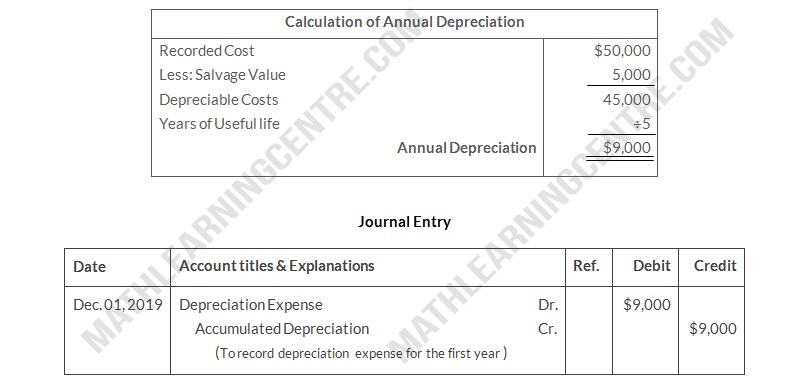On January 1, 2019, Evers Company purchased the following two machines for use in its production process.
 Machine A: The cash price of this machine was \$48,000. Related expenditures included: sales tax \$1,700, shipping costs \$150, insurance during shipping \$80, installation and testing costs \$70, and \$100 of oil and lubricants to be used with the machinery during its first year of operations. Evers estimates that the useful life of the machine is 5 years with a \$5,000 salvage value remaining at the end of that time period. Assume that the straight-line method of depreciation is used. Machine B: The recorded cost of this machine was \$180,000. Evers estimates that the useful life of the machine is 4 years with a \$10,000 salvage value remaining at the end of that time period

### Instructions

1. Prepare the following for Machine A
1. The journal entry to record its purchase on January 1, 2019
2. The journal entry to record annual depreciation at December 31, 2019.
2. Calculate the amount of depreciation expense that Evers should record for Machine B each year of its useful life under the following assumptions.
1. Evers uses the straight-line method of depreciation.
2. Evers uses the declining-balance method. The rate used is twice the straight-line rate.
3. Evers uses the units-of-activity method and estimates that the useful life of the machine is 125,000 units. Actual usage is as follows: 2019, 45,000 units: 2020, 35,000 units: 2021, 25,000 units: 2022, 20,000 units.
3. Which method used to calculate depreciation on Machine B reports the highest amount of depreciation expense year 1 (2019)? The highest amount in year 4 (2022)? The highest total amount over the 4-year period?

### Solution

a.
For Machine A
1.2.b.
For Machine Bc.
--Highest depreciation in Year 1 (2019) = Declining balance \$ 90000
--Highest amount in Year 4 (2022) = Straight Line
--Highest total amount over 4 year period = No matter which of the three methods is used. Total depreciation of each methods are same at 4 years period.